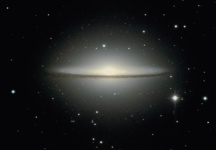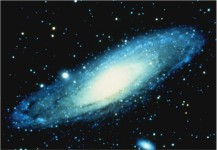# The universe - vast beyond measure.

• One estimate (1976) of the total mass of the universe was 1041kg.
• A more recent estimate of the total mass of the universe is 1053kg.
• None of us know the true size of the universe.
• As far as humans are concerned, the universe is effectively infinite in size.

Q1: How did all the matter get there?
Q2: Where did all the energy come from, to create the universe?• According to the energy-mass equation, E=mc2, the energy required to form matter is the mass (in kg) multiplied by the speed of light squared. The speed of light ("c") is 3 x 108 metres/second. Thus, the energy required to form the universe is 1053 x 9 x 1016 = 9 x 1069 Joules.
• Evolution cannot explain where all the matter originally came from, nor the energy to form it all.
• The Hubble space telescope was launched in 1990 to gain a better understanding of the universe.
They decided to take a photo of "empty" deep space, just to see if anything was there.
After processing the time delay image, they were surprised to see what appeared to be "empty" deep space, was actually jammed packed full of stars and galaxies which filled the entire photo frame.
You can see those photos on the Hubble Space Telescope website.

Solution: Only the infinite power source (God), can form an infinite universe.